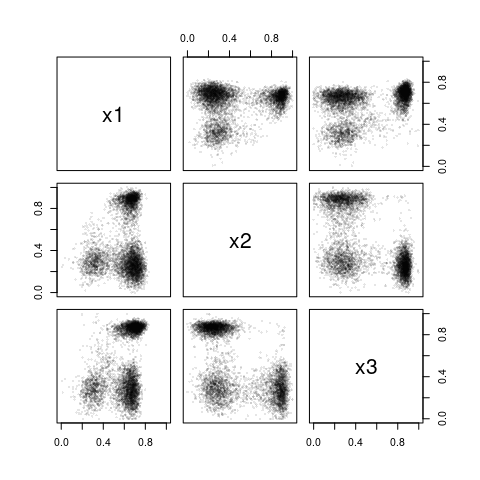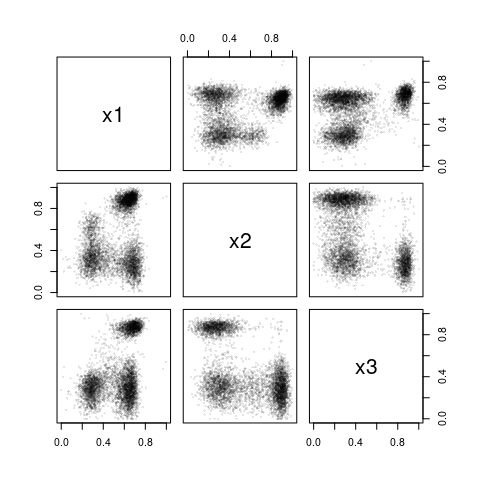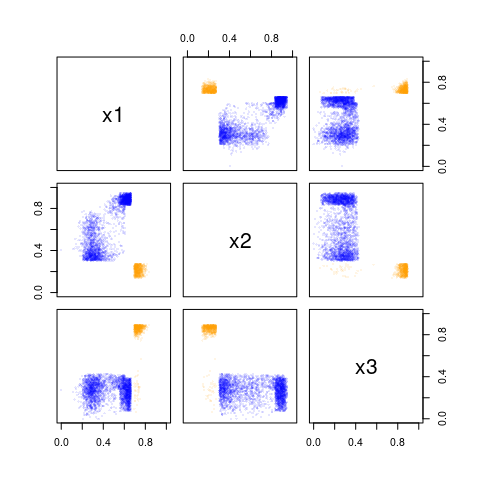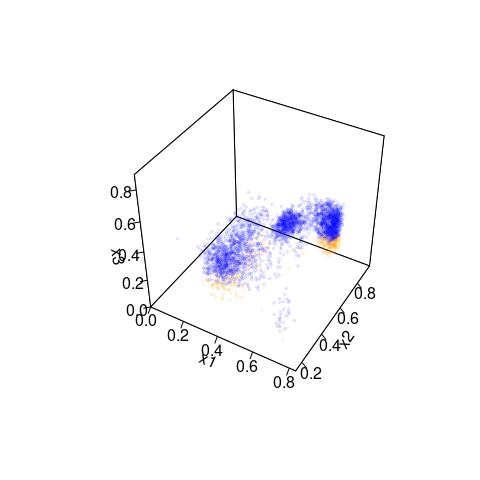# Using prim to estimate highest density difference regions

#### 05 February 2021

The Patient Rule Induction Method (PRIM) was introduced by Friedman and Fisher (1999). It is a technique from data mining for finding interesting’ regions in high-dimensional data. We start with regression-type data (X1, Y1), …, (Xn, Yn) where Xi is d-dimensional and Yi is a scalar response variable. We are interested in the conditional expectation function

m(x) = E (Y | X = x).

In the case where we have 2 samples, we can label the response as a binary variable with

Yi = 1 if Xi is in sample 1

or

Yi = -1 if Xi is in sample 2.

Then PRIM finds the regions where the samples are most different. Here we have a positive HDR (where sample 1 points dominate) and a negative HDR (where sample 2 points dominate).

We look at a 3-dimensional data set quasiflow included in the prim library. It is a randomly generated data set from two normal mixture distributions whose structure mimics some light scattering data, taken from a machine known as a flow cytometer.

library(prim)
data(quasiflow)
yflow <- quasiflow[,4]
xflow <- quasiflow[,1:3]
xflowp <- quasiflow[yflow==1,1:3]
xflown <- quasiflow[yflow==-1,1:3]

We can think of xflowp as flow cytometric measurements from an HIV+ patient, and xflown from an HIV– patient.

pairs(xflowp, cex=0.5, pch=16, col=grey(0,0.1), xlim=c(0,1), ylim=c(0,1))pairs(xflown, cex=0.5, pch=16, col=grey(0,0.1), xlim=c(0,1), ylim=c(0,1))There are two ways of using prim.box to estimate where the two samples are most different (or equivalently to estimate the HDRs of the difference of the density functions). In the first way, we assume that we have suitable values for the thresholds. Then we can use

qflow.thr <- c(0.38, -0.23)
qflow.prim <- prim.box(x=xflow, y=yflow, threshold=qflow.thr, threshold.type=0)

An alternative is compute PRIM box sequences which cover the range of the response variable y, and then use prim.hdr to experiment with different threshold values. This two-step process is more efficient and faster than calling prim.box for each different threshold. We are happy with the positive HDR threshold so we can compute the positive HDR directly:

qflow.hdr.pos <- prim.box(x=xflow, y=yflow, threshold=0.38, threshold.type=1)
summary(qflow.hdr.pos)
#>              box-fun   box-mass threshold.type
#> box1     0.551879699 0.05808875              1
#> box2*   -0.003431327 0.94191125             NA
#> overall  0.028825996 1.00000000             NA

On the other hand, we are not sure about the negative HDR thresholds. So we try several different values for threshold.

qflow.neg <- prim.box(x=xflow, y=yflow, threshold.type=-1)
qflow.hdr.neg1 <- prim.hdr(qflow.neg, threshold=-0.23, threshold.type=-1)
qflow.hdr.neg2 <- prim.hdr(qflow.neg, threshold=-0.43, threshold.type=-1)
qflow.hdr.neg3 <- prim.hdr(qflow.neg, threshold=-0.63, threshold.type=-1)

After examining the summaries and plots,

summary(qflow.hdr.neg1)
#>            box-fun   box-mass threshold.type
#> box1    -0.6767677 0.05188679             -1
#> box2    -0.3109244 0.05197414             -1
#> box3    -0.2778316 0.09023410             -1
#> box4    -0.2815199 0.05057652             -1
#> box5*    0.1580895 0.75532844             NA
#> overall  0.0288260 1.00000000             NA

we choose qflow.hdr.neg1 to combine with qflow.hdr.pos.

qflow.prim2 <- prim.combine(qflow.hdr.pos, qflow.hdr.neg1)
summary(qflow.prim2)
#>            box-fun   box-mass threshold.type
#> box1     0.5518797 0.05808875              1
#> box2    -0.6767677 0.05188679             -1
#> box3    -0.3109244 0.05197414             -1
#> box4    -0.2778316 0.09023410             -1
#> box5    -0.2815199 0.05057652             -1
#> box6*    0.1252819 0.69723969             NA
#> overall  0.0288260 1.00000000             NA

In the plot below, the positive HDR is coloured orange (where the HIV+ sample is more prevalent), and the negative HDR is coloured blue (where the HIV- sample is more prevalent)

plot(qflow.prim2, x.pt=xflow, pch=16, cex=0.5, alpha=0.1)or equivalently as a 3D scatter plot.

plot(qflow.prim2, x.pt=xflow, pch=16, cex=0.5, alpha=0.1, splom=FALSE, colkey=FALSE, ticktype="detailed")`